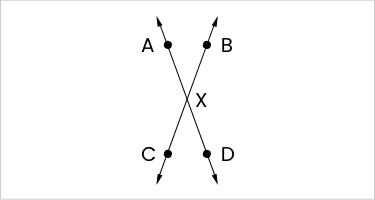### Still have math questions?

Geometry
Question

In the figure below, if $$m\angle\text{AXC}=10x$$, then $$m\angle\text{BXD}=?$$.$$10x$$

$$360-10x$$

$$90-10x$$

$$180-10x$$

$$10x$$